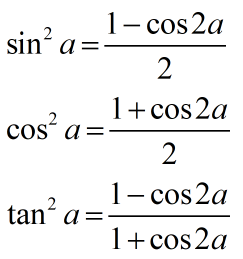Request a Tool

Power Reduction Identity Calculator

Power Reduction Identity Calculator is use to calculate reduction of power from sin, cos and tan.

Output

Power Reduction Identity
0

Formula• sina^2 = Power reduce for sin
• cosa^2 = Power reduce for cos
• tana^2 = Power reduce for tan
• a = Value of angle

Defination / Uses

Trigonometric identities that allow us to recast trigonometric formulas raised to a power in terms of smaller trigonometric expressions are known as power reducing identities.

We'll look at the power lowering formulas that connect the squares of the trigonometric functions (sin2(x), cos2(x), and tan2(x)) to the cosine of the angle doubled (i.e., using the cos(2x) identity). It's a simple technique to switch between different powers of trigonometric functions, from smaller to larger or vice versa. The method of employing a reducing power function to evaluate the squared value of the three basic trigonometric functions (sin, cos, and tan) is known as power reduction. Solving the second and third versions of the cosine double-angle and half-angle formulas yields the power reduction formulas.